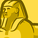# New to Qlik Sense

If you’re new to Qlik Sense, start with this Discussion Board and get up-to-speed quickly.

Announcements
Qlik Insider: SaaS capabilities for Data Integration and Analytics, May 25th! Register Now
cancel
Showing results for
Did you mean:Contributor

## Variable depending on other variable

Hi everyone,

fist of all, let me introduce myself. I'm head of Controlling department and an expert of excel and vba for excel, recently I have discovered Qlik sense and now I'm a beginner. Let me introduce you my problem.

I have a chart with a variable in the measure

=Sum(\$(vSG&A))

This variable is automatically changed by an extension called "Variable", which let me change the measure by clicking buttons.

For example, if I click in SG&A button, this extension introduce in the variable vSG&A the value '[Selling exp.] + [GA exp.]' and the chart shows this addition, if I select Selling Expenses button, the variable introduce only '[Selling exp.]' and the chart is updated with this info.

It works perfectly.

Well, the problem is that I would like to update the reference line with my forecast too. But the Forecast figures are in other measures called [Selling exp. FC]. I have created a new variable and introduced this function in the reference line the next:

=Sum({\$<[Month_Key]={\$(vForecast)}>}\$(vSG&A FC))

And the key for me is the definition of this new variable (vSG&A FC). I want that depending on the value of vSG&A the vSG&A FC has one or other value. ¿Is it possible?

I have written the next in the definition of the variable but it's not working:

=if( \$(vSG&A)='[Selling exp.] + [GA exp.]','[Selling exp. FC] + [GA exp. FC]',

if( \$(vSG&A)='[Selling exp.]','[Selling exp. FC]',

))

What's wrong??